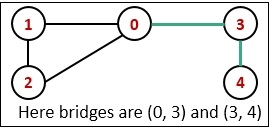# Bridges in a Graph

An edge in an undirected graph is said to be a bridge, if and only if by removing it, disconnects the graph, or make different components of the graph.In a practical approach, if some bridges are present in a network when the connection of bridges is broken, it can break the whole network.

## Input and Output

Input:
The adjacency matrix of the graph.

0 1 1 1 0
1 0 1 0 0
1 1 0 0 0
1 0 0 0 1
0 0 0 1 0

Output:
Bridges in given graph:
Bridge 3--4
Bridge 0--3

## Algorithm

bridgeFind(start, visited, disc, low, parent)

Input − The start vertex, the visited array to mark when a node is visited, the disc will hold the discovery time of the vertex, and low will hold information about subtrees. The parent will hold the parent of the current vertex.

Output − print if any bridge is found.

Begin
time := 0       //the value of time will not be initialized for next function calls
mark start as visited
set disc[start] := time+1 and low[start] := time + 1
time := time + 1

for all vertex v in the graph G, do
if there is an edge between (start, v), then
if v is visited, then
parent[v] := start
bridgeFind(v, visited, disc, low, parent)
low[start] := minimum of low[start] and low[v]

if low[v] > disc[start], then
display bridges from start to v
else if v is not the parent of start, then
low[start] := minimum of low[start] and disc[v]
done
End

## Example

#include<iostream>
#define NODE 5
using namespace std;

int graph[NODE][NODE] = {
{0, 1, 1, 1, 0},
{1, 0, 1, 0, 0},
{1, 1, 0, 0, 0},
{1, 0, 0, 0, 1},
{0, 0, 0, 1, 0}
};

int min(int a, int b) {
return (a<b)?a:b;
}

void bridgeFind(int start, bool visited[], int disc[], int low[], int parent[]) {
static int time = 0;
visited[start] = true;               //make the first vertex is visited
disc[start] = low[start] = ++time;  //initialize discovery time and the low time

for(int v = 0; v<NODE; v++) {
if(graph[start][v]) {   //for all vertex v, which is connected with start
if(!visited[v]) {
parent[v] = start;    //make start node as parent
bridgeFind(v, visited, disc, low, parent);

low[start] = min(low[start], low[v]);    //when subtree from v is connected to one of parent of start node
if(low[v] > disc[start])
cout << "Bridge " << start << "--"<<v<<endl;
} else if(v != parent[start])    //update low of start for previous call
low[start] = min(low[start], disc[v]);
}
}
}

bool bridges() {
bool *vis = new bool[NODE];
int *disc = new int[NODE];
int *low = new int[NODE];
int *parent = new int[NODE];

for(int i = 0; i<NODE; i++) {
vis[i] = false;    //no node is visited
parent[i] = -1;    //initially there is no parent for any node
}

for(int i = 0; i<NODE; i++)
if(!vis[i])    //if any node is unvisited, the graph is not connected
bridgeFind(i, vis, disc, low, parent);
}

int main() {
cout << "Bridges in given graph:"<<endl;
bridges();
}

## Output

Bridges in given graph:
Bridge 3--4
Bridge 0--3